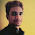## 26 May 2015

### Answers to Aptitude and Numerical questions paper-1

You are here UCEED >> Solution to Numerical questions practice paper - 1
--------------------------------------------------------------------------------------------
In this post, I have covered answers/solutions for the numerical questions that I've included in my previous post - Numerical and aptitude questions for UCEED  for your reference. I have also added some additional aptitude questions at the end. Similar pattern might appear in your UCEED exam.
--------------------------------------------------------------------------------------------

1)
Suppose a water tank is in the shape of a right circular cylinder is 20 meter long and 10 meter in diameter. How much sheet metal will be used in its construction. Picture shown below

Metal sheet required = surface area of the cylinder (PI*d*h)+ area of the two circles that are used to close the water tank (2*PI*sqrt(r))

= PI*10*20 + 2*PI*sqrt(5)
= 250*PI

2)
A piece of wire of 42 cm long is bent into the shape of a rectangle whose width is twice its length. Find the dimensions of the rectangle.

Answer : L = 7, W = 14

3)
A 10 meter pole casts a shadow of 5 meter. How tall is a tree with a shadow of 12 meter ?

4)
A man runs round a circular field of radius 14 meter at the speed of 88 m/hr. What is the time taken by the man to take twenty rounds of the field ? (take PI = 22/7)

5)
A wire can be bent in the form of a circle of radius 7cm. If it is bent in the form of a square, then its area will be ? (take PI = 22/7)

6)
A building of height 6 meter casts a shadow of 5 meter, then what will be the length of the stand post which casts 10 meter shadow

7)
A rectangular plot measuring 90 meters by 50 meters is to be enclosed by wire fencing. If the poles of the fence are kept 5 meters apart. How many poles will be needed for that ?

Perimeter of the rectangle is 90+50+90+50 = 280, which when divided by 5 (because for every 5 meter a fencing pole is there) we get the number of poles
i.e. 280/5 = 56

8)
If one side of a square is doubled in length and the adjacent side is decreased by two centimeters, the area of the resulting rectangle is 96 square centimeters larger than that of the original square. Find the dimensions of the rectangle.

Answer : L = 24 cm, B = 10 cm

9)
Next 2 numbers in the series 4,8,9,27 .....

Answer : its 4 sqr and 4 cube i.e 16,64

10)
A metallic sphere of radius 3 cm is melted and recast into the shape of a cylinder of radius 3 cm, Find the height of the cylinder.

11)
Metallic spheres of radii 6 cm, 8 cm and 10 cm are melted to form a single solid sphere. Find the radius of the resulting sphere

12)
A 20m deep well with diameter 7 m is dug and the earth from digging is evenly spread out to form a platform 22m by 14m. Find the height of the platform
(You may use calculator for this particular question, the main thing you need to understand is the concept )

13)
A rectangular sheet of dimensions 44 cm X 18 cm is rolled along its length and a cylinder is formed. Find the volume of the cylinder.

The given sheet is a rectangle with L=44cm and b=18 cm, so when we roll/fold along its length, it does mean that the new obtained cylinder will have height = breadth of the sheet (i.e 18 cm) and length of the sheet will now be the perimeter or circumference of the new cylinder. right ???

so,
h = 18 cm, and
2*PI*R = 44 cm which will give R = 7 cm,

now the volume of cylinder is PI*sqr(R)*h

14)
An ant crawls from one corner of a room to a diametrically opposite corner along the shortest possible path. If the dimensions of a room are 3X3X3, what distance does the ant cover ?

If the sides of a cube are say a,b,c, then the shortest distance traveled by the ant from one bottom corner to diagonally opposite top corner is the minimum of

Sqrt[(a^2+b^2) + c^2], Sqrt[(b^2+c^2) + a^2], Sqrt[(a^2+c^2) + b^2]

In reality if u assume the box to be opened, then the two adjacent faces (on which the ant travels) will flatten to a rectangle shape with the length as (a+b) and width/height as c, now the shortest distance is the straight line joining the two points, i.e the diagonal which is obtained by using Pythagoras theorem.

The thing is the ant will crawl along from bottom point to the top diagonal by crawling along its wall. The ant will travel along two side walls, so instead of viewing that in 3D, We assume that the side wall in unfolded to the same plane as the first to from a 2D rectangle of bottom length = length of first wall + Length of second wall = a+b , and height equal to actual height of the solid cube. The shortest distance will now be the diagonal of he new rectangle.

15)
There are 2 trees in a garden (say A and B) and on both the trees, there are some birds. The birds of tree A says to the bird of tree B that if one of you comes to our tree, then our population will be the double of yours. The birds of tree B tell to the birds on tree A that if one of you comes here, then our population will be equal to that of yours. How many birds are there in trees A and B ?

Let the no. of birds on tree A be "x"
and the no. of birds on tree B be "y"
so as per the first statement if one bird from B comes to tree A then no. of birds on A will be double of B". so
(x+1) = 2*(y-1)

as per the second statement "if one bird joins tree B from A, then the number of birds on both will be same ". So

(y+1) = (x-1)

now solve the above two equations for x and y.

16)
A cylinder 108 cm high has a circumference of 24 cm. A string makes exactly 6 complete turns round the cylinder while its two ends touch the cylinder's top and bottom. How long is the string in cm?

The string is making 6 complete turns of height 108 cm, so for one complete turn it will cover 108/6 = 18 cm height
now assume we have a cylinder of height 18 cm and circumference 24 cm, if you unfold/unwrap the cylinder into a plain sheet, we will get a rectangle of width = height of cylinder and length of rectangle = circumference of the cylinder

Now the shortest length of the string is the diagonal of the rectangle which can be obtained by Pythagoras theorem i.e sqrt(18^2 + 24^2)
which will come to be 30 cm
so for a single turn it is 30cm, for the total 6 turns, length = 30*6 = 180cm

17)
A creeper plant is climbing up and around a cylindrical tree trunk in a helical manner. The tree trunk has a height of 720 cm and a circumference of 48 cm.
If the creeper covers a vertical distance of 90 cm in one complete twist around the tree trunk, what is the total length of the creeper? (You may calculator for now, concept is important)

Applying the same concept as we applied for the prev question, we wil get the solution
It's already given that for one complete turn of the creep, the height is 90 cm, so just like previous problem we will unfold the cylinder to a rectangle of dimensions L = 48 cm and b = 90 cm
Finding the diagonal as sqrt(48^2 + 90^2) = 102 cm; which is the length of the creep for a single turn
Total number of complete turns = 720/90 = 8
So, the total length = 102*8 = 816 cm

18)
There are 6 people in a room. They shake each other's hands once and only once. How many handshakes are there altogether?

19)
Your teacher has a total of 16 chalks. When a chalk reduces to 1/4 of its original size, it gets too small for her to hold for writing and hence she keeps it aside. But your teacher hates wasting things and so, when she realizes that she has enough of these small pieces to join and make another chalk of the same size, she joins them and uses the new chalk stick. If she uses one chalk each day, how many days would the 16 chalks last?

Note the working as below

1.since the teacher uses one chalk a day, so for sixteen days 16 fresh chalks she uses

2. as stated in problem, every chalk leaves 1/4 th piece a day, so for 16 days she will have 16 pieces of 1/4th which she will join to get 4 chalks, got ? These four chalks she will use four more days

3. As usual for four days, the number of 1/4 piece left is 4, which she joins to get a single chalk

4. That chalk she will use the next day

So, summing them, we have 16+4+1 = 21 days

20)
A snail can crawl 5 meters in 5 min, what will be the total distance covered by a group of 5 snails starting at the same time in 5 min ?

21)
Somu and Bhanu each have a collection of tennis balls. Somu said that if Bhanu would give him 4 of his balls they would have an equal number; but, if Somu would give Bhanu 4 of his balls, Bhanu would have 2 times as many balls as Somu. How many balls does Bhanu have?

The solution goes like this

Let the no. of balls with Somu be "x"
and the no. of balls with Bhanu be "y"
so as per the first statement "if Bhanu gives four balls to Somu, then both will have equal" so
(x+4) = (y-4)

as per the second statement "if Somu gives four balls to Bhanu, then Bhanu will have double than Somu ". So

(y+4) = 2*(x-4)
now solve the above two equations for x and y.

22)
A tennis championship is played on a knock-out basis, i.e., a player is out of the tournament when he loses a match.
• How many players participate in the tournament if 85 matches are totally played ?
• How many matches are played in the tournament if 17 players totally participate?

23)
There are five different houses A to E. A is to the is to the right of B and E is to the left of C and right of A. B is to the right of D. Which of the houses is in the middle ?

24)
Ram noticed in a cycle stand that there were a total of 14 bicycles and tricycles. If the total number of wheels was 35, how many tricycles were there?

25)
Suppose 6 monkeys take 6 minutes to eat 6 bananas, how many minutes would it take
• 3 monkeys to eat 3 bananas
• 24 monkeys to eat 24 bananas

6 monkeys - 6 min. - 6 bananas
1 monkey - 6 min. - 6/6 bananas ( = 1 banana)
multiply by 3
3 monkeys - 6 min, - 3*1 banana
simlary, multiply by 24
24 monkeys - 6 min, - 24*1 banana

Note that all the monkeys eat simultaneously, so, 6 monkeys together eat 6 bananas in 6 minutes, that means, a monkey can eat one banana in 6 min.
so, in the same time of 6 min, 'n' monkeys can eat 'n' bananas.

26)
A snail creeps 8 ft up a wall during the daytime. After all the labor it does throughout the day, it stops to rest a while... but falls asleep!! The next morning it wakes up and discovers that it has slipped down 5 ft while sleeping. If this happens every day, how many days will the snail take to reach the top of a wall 29 ft in height?

first day, the snail goes 8ft, but at night, it slips to (8-5) = 3ft
second day, it has to start at 3 ft and by the end of the day, it will have reached 3+8 =11ft, but at night it will slip to 5ft, thus totalling to 11-5 = 6ft
So, every at the end of everyday it will go 3ft height

At the end of 7th day, it would have climbed till, (3*6 + 8) = 26 ft, but at 7th day night, it might have fall down to 26-5 = 21 ft

on 8th day, it will reach 21ft + 8ft = 29ft, by then it has reached the top and so it doesn't have to slip down. So, the answer is 8 days

27)
A 24 cm x 24 cm square metal plate needs to be fixed by a carpenter on to a wooden board. The carpenter uses nails all along the edges of the square such that there are 25 nails on each side of the square. Each nail is at the same distance from the neighbouring nails. How many nails does the carpenter use?

28)
A block of wood in the form of a cuboid 6cm × 4cm × 4cm has all its six faces painted pink. If the wooden block is cut into 96 cubes of 1cm × 1cm × 1cm, how many of these would have pink paint on them?

Only those cubes at the surface of the cube will have the colored surface.
there will be 4 surfaces with sides 6cm x 4cm
and two surfaces of sides 4cm x 4cm

So, the total number of 1 X 1 cubes will be = 6*4*4 + 4 *4*2 = 128

But out of this, some cubes will be shared by adjacent surfaces, leke all the cubes at the eight corners of the cuboid will be shared by 3 surfaces (2 times counted extra for each corner cube), so for all these eight corners, we have to remove = 8*2 = 16
Also, there will be cubes at the sides/edges of the cuboid, which will be shared by two adjacent surfaces, like that there will be - (6-2)*4 + (4-2)*2*4 = 32

So, total will be 128 - 16-32 = 80

You can check detailed explanation for similar question in Question 2 of CEED 2015 answer key with solutions

29)

In a classroom of 100 students, 40 students feel alert, while 60 students feel bored, if 50% of the alert students turn bored. After that, 50% of the bored students turn alert, what is the final number of students who feel alert and bored ?

b. No change - 40 alert, 60 bored
c. 60 alert, 40 bored (ans)

Explanation :

Initial step :

Bored = 60

Step-1 :

50% of the alert students turns bored. so,
Alert = 40 - 40*0.5 = 20
Bored = 60 + 20(from alert) = 80

Step-2:

50% of the bored students turns alert
Bored = 80 (from previous step) - 80*0.5 = 40

Alert = 20 (from previous step) + 40 = 60

--------------------------------------------------------------------------------------------

Hope the above questions gave you some idea as well as helped you as part of your practice.

Like stuffyoulook Facebook page to get updated with latest posts!

1.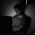Could you explain 1st question. Do we have to find the total surface area of that

1.I explained the answer above, you may check now

2.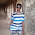could you explain 12th question

3.can i get explanation for 22,24,25,26,27,28 plz sir can

4.u can find those answers in other mock tests especially most are answered as comments in this post

GA practice exercise for everyday study

2.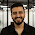thank you for these wonderful questions.
in quest 7:(no of poles)
you have considerd the linear length of the plot and answered 280/5=56 poles.
but sir. as it is a closed loop. the poles will repeat at the corners.
ie: length 90m so 90/5=18 poles x 2= 36poles (including corner ones)
breadth 50m so 50/5=10poles x 2=20poles - 4poles(corners already included above) =16poles.
therefor its total will only be (36+16)poles= 52poles.

1.Consider a straight line of length 10m, As per your explanation, the number of poles should be 10/5 = 2 no's right ?
Let's check the poles required. Now starting from the start point (which is zero) for the first five meter - 1 pole, for the next five meter - one more pole, if you consider the pole at the zero location too, then the total no. of poles for this straight line is 3 and not 2! which is (10/2 + 1)

Now, apply this logic, you will get the answer.

Bottom horizontal line = 1 + 18
right vertical line = 10 (1 pole already covered by the bottom line)
top horizontal line = 18 (1 pole already covered by right vertical line)
left vertical line = 10 - 1 (1 pole is already occupied at the zero position while calculating bottom horizontal line)

2.thums up!. thank you for the clarified explanation. i got 56. :)

3.Plz tell me the solution of first question

1.I explained the answer above, you may check now

4.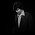Plz explain question 26

5.Sir in 26 ques i think ans should be 10 days

6.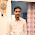**Just a suggestion:** Use Mathjax to represent Mathematical formulas in your blog and it will be whole lot easier to understand for everyone.

1.Thank u for the suggestion, will look into that for future posts.

7.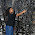i think in question 2 the lenght will be 14 n width will be double of it which is 28. and 28+14= 42????? am i right?

1.hey, actually, it's a rectangle, so its perimeter = 2L + 2W
and so, L should be 7, and W should be 14, so that 2L+2W = 42. got it ?

8.can you plz explain ques 4 n 5?

1.q4)
Since the radius of the circle is 14m, the total len, i.e. circumference around = 2*PI*r = 2*22/7*14 = 88m
given, speed is 88m/hr, it mean for every 1 hour, the distance covered is 88m,
for 20 such laps, the time taken is hence 20*1hr = 20hr, and the total lap distance = 88*20 = 1760m

2.q5)
initially the wire is bent to circle, so the total len of the wire will be its circumference, = 2*PI*r = 2*22/7*7 = 44 units

now, whether they are bent to rectangle/any shape, doesn't matter, coz same quantity of wire will be used, so using 44 units of wire, if its bent to a square of side a, then the total len (perimeter) is 4*a,
but we know total len = 44, so 4*a = 44, implies a = 11,
now, the area of the square = square(a) = 11^2 = 121

9.in q 19 shouldn't the answer be 21 days?

10.how did u get 8 in q 26 sir,by my method i am getting 9 days.please explain.

1.it's better if u explain so that we can chk ur solution

2.hello sir,
here is a solution for question no. 26 if its right plz rply
in 1 day a snail climbs 8 ft nd moves dwn 5ft so total it climbed is 3 ft each day it climbs 3 ft so acc to tht it should take 10 days

3.I've updated the answer above, u all can chk now

11.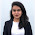sir,
can you please provide explanation for Q. 25 and Q.28 ?
And I have solved sample papers for CEED and UCEED provided by your blog. are there any other papers I can solve for UCEED ?

1.I've updated above, u can chk,

solving only previous paper will not help you to the full. They r just a way to tell u about the pattern and the syllabus that they cover. You need to go thru the theory part (esp GA) as well as solve similar questions (for math n apti part). U better start studying the available resources in resource page!

12.answer of Q 28 should be 51.
as here we are talking about the no. of cubes not the faces.
Therefore,
total no. of unit cubes in 6*4*4=96 (small cubes 1*1*1)
Now every surface is painted.
so, by removing cube of the dimension 5*3*3 we get the no. of cubes left unpainted. because only outside surface is painted and unit dimension of small cubes is 1 unit.
(6-1)*(4-1)*(4-1)=(5)*(3)*(3)= 45 unit cubes

No. of unit cubes painted= 96-45= 51

13.I think the answer of Question no. 7 would be 52 because we are counting the poles at the corner twice so 56-4=52

14.In Q.28 can't we just decrease two units from from the dimensions given? So that we find how many cuboids are there with no paint. And then subtract it from 96, i.e the total number of cubes.

like, 6 becomes 4, 4 becomes 2 and the other 4 becomes 2. so total voln. is 16 unit cube. Which means there are 16 unpainted cuboids.
So, 96-16= 80.

1.Literally the same as above, but in diff way. Yeahc this will be good too. After all, math probs have several ways of solving. That’s its beauty.
!

15.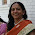Sir, shouldn't the answer of question no. 6 be 11?
1.